# Unified Council - UIMO PDF Sample Papers for Class 7

Resources:

Class 7 sample paper & practice questions for Unified International Mathematics Olympiad (UIMO) level 1 are given below. Syllabus for level 1 is also mentioned for these exams. You can refer these sample paper & quiz for preparing for the UIMO exam.Q.1 Q.2 Q.3 Q.4 Q.5 Q.6 Q.7 Q.8 Q.9 Q.10
 Q.1 Which of the following has the top, side and front as triangle? a)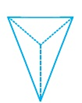b)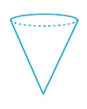c)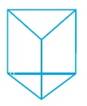d)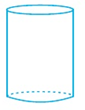Q.2 In fig, AB|| CD, ∠APQ=50° and ∠PRD = 130°. Find the value of ∠QPR.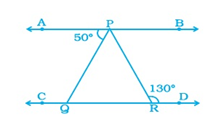a) 130° b) 50° c) 80° d) 30°
 Q.3 In the figure, POR is a straight line.  Find the value of a.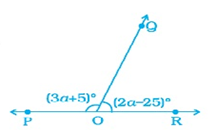a) 32° b) 55° c) 40° d) 42°
 Q.4 Choose the correct option. If the radius of a circle is tripled, then the area becomes ___. a) 3 times b) 6 times c) 30 times d) 9 times
 Q.5 225% is equal to what ratio? a) 4: 9 b) 3: 4 c) 2: 3 d) 9: 4
 Q.6 Choose a figure which would most closely resemble the unfolded form of Figure (Z).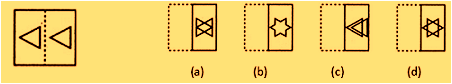a) a b) b c) c d) d
 Q.7 Name the solid that has two opposite identical faces and other faces as parallelogram. a) Pyramid b) Prism c) Sphere d) Cone
 Q.8 What is the circumference of a circle with diameter 5 cm? a) 3.14 cm b) 31.4 cm c) 15.7 cm d) 1.57 cm
 Q.9 Three candidates contested an election and received 1136, 7636 and 11628 votes respectively. What percentage of the total votes did the winning candidate got? a) 58% b) 56% c) 57% d) 55%
 Q.10 A lends Rs. 3500 to B at 10% p.a. and B lends the same sum to C at 11.5% p.a. Find the gain of B (in Rs.) in a period of 3 years. a) Rs. 154.50 b) Rs. 155.50 c) Rs. 157.50 d) Rs. 156.50Sample PDF of Unified Council - Unified International Mathematics Olympiad (UIMO) PDF Sample Papers for Class 7: